Courses

# Torsion of Shafts and Pressure Vessels Civil Engineering (CE) Notes | EduRev

## Civil Engineering (CE) : Torsion of Shafts and Pressure Vessels Civil Engineering (CE) Notes | EduRev

The document Torsion of Shafts and Pressure Vessels Civil Engineering (CE) Notes | EduRev is a part of the Civil Engineering (CE) Course Topic wise GATE Past Year Papers for Civil Engineering.
All you need of Civil Engineering (CE) at this link: Civil Engineering (CE)

Q.1 A closed thin-walled tube has thickness, t, mean enclosed area within the boundary of the centerline of tube's thickness, Am and shear stress, τ. Torsional moment of resistance T, of the section would be    [2019 : 1 Mark, Set-ll]
(a) 2τAmt
(b) 4τAmt
(c) τAmt
(d) 0.5τAmt
Ans.
(A)
Solution: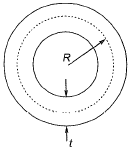Shear stress,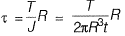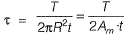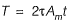Q.2 A solid circular beam with radius of 0.25 m and length of 2 m is subjected to a twisting moment of 20 kNm about the z-axis at the free end, which is the only load acting as shown in the figure. The stress component τry at Point ‘ M ’ in the cross- section of the beam at a distance of 1 m from the fixed end is    [2018 : 1 Mark, Set-I]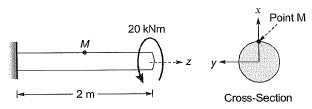(a) 0.0 MPa
(b) 0.51 MPa
(c) 0.815 MPa
(d) 2.0 MPa
Ans.
(A)
Solution: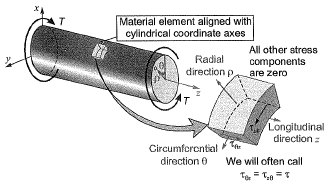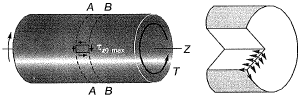The only non-zero stresses are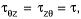if θ is 900 then θ = y
Hence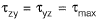= 16T/πd3 = 0.815 MPa
But in rest of the planes shear stresses are zero, hence,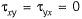Q.3 A hollow circular shaft has an outer diameter of 100 mm and inner diameter of 50 mm. If the allowable shear stress is 125 MPa, the maximum torque (in kN-m) that the shaft can resist is _____.    [2017 : 2 Marks, Set-II]
Solution: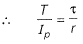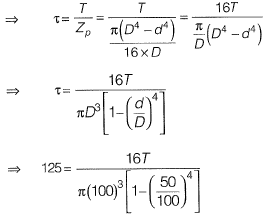⇒ T = 23 kNm

Q.4 A solid circular shaft of diameter d and length L is fixed at one end and free at the other end. A torque T is applied at the free end. The shear modulus of the material is G. The angle of twist at the free end is    [2010 : 1 Mark]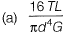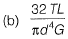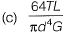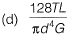Ans.
(b)
Solution:
Angle of twist is given by,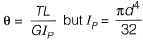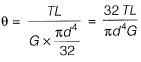Offer running on EduRev: Apply code STAYHOME200 to get INR 200 off on our premium plan EduRev Infinity!

69 docs

,

,

,

,

,

,

,

,

,

,

,

,

,

,

,

,

,

,

,

,

,

;